# 二、数据导入、存储

## 1. 文本格式

pandas提供了一些用于将表格型数据读取为DataFrame对象的函数。以下

csv文件就是一个以逗号分隔字段的纯文本文件，用于测试的文件是本身是一个Excel文件，需要修改一下扩展名，但是简单的修改后缀名不行，还需要将字符编码改变为utf-8，因为默认的是ASCII，否则是会报错的。然后就可以通过read_csv将它读入到一个

DataFrame：

import pandas as pd
import numpy as np

data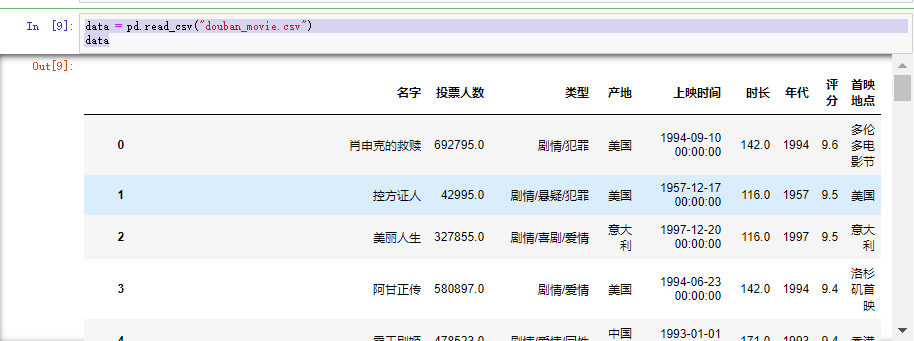需要注意的是：

data = pd.read_table("douban_movie.csv",sep=',')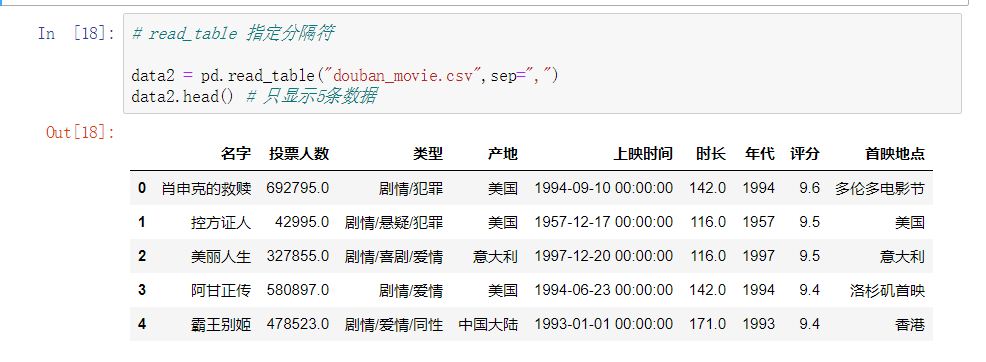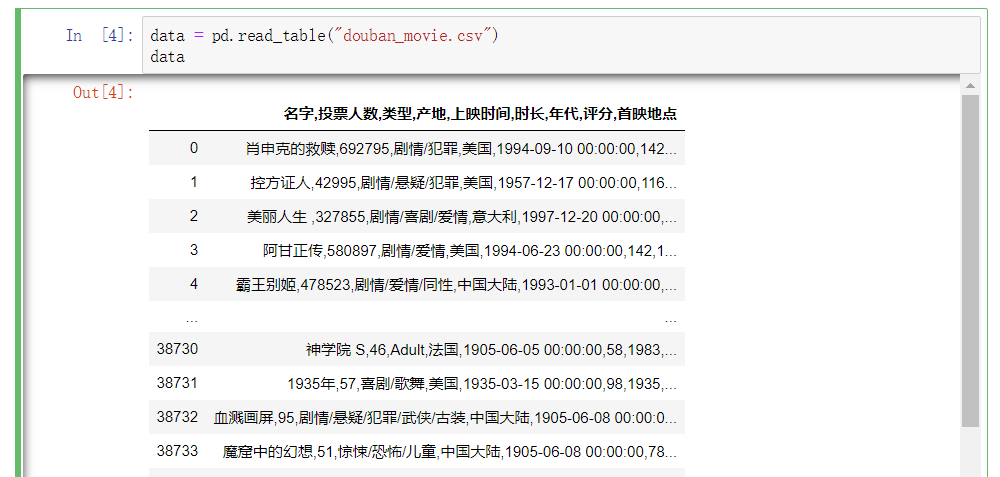### （3）指定列名

pandas可以帮助我们自动分配列名，也可以自已指定列名

## 2. 二进制

### （1）pickle

import pandas as pd
df.to_pickle("E:/Test/df_pickle")


### （2）HDF5

HDF5主要用于存储大量的科学数组数据。以C库的形式提供，并且有许多其他语言的接，列如：java、julia、当然还有我们的Python。

HDF5中的HDF代表分层数据格式，每个HDF5文件可以存储多个数据集并且支持元数据

import pandas as pd
import numpy as np

frame = pd.DataFrame({'a':np.random.randn(100)})

frame.to_hdf('E:/jupyter/pandas_test/mydata.h5','obj3',format='table')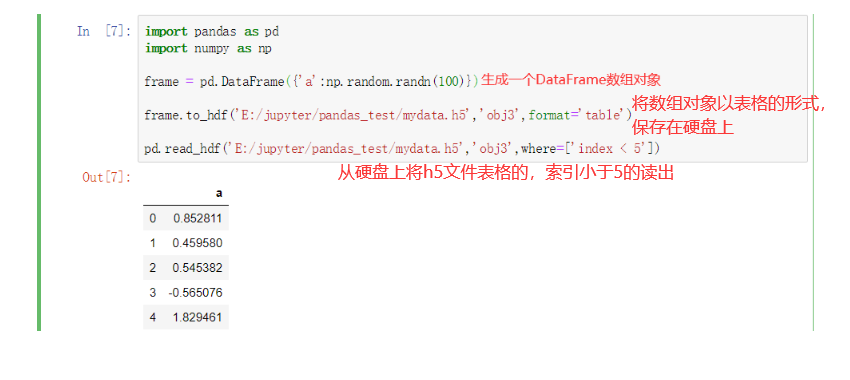## 3.Web API

url = "https://api.github.com/repos/pandas-dev/pandas/issues"
resp = requests.get(url)
data = resp.json()
# 因为data中的每个元素都是一个字典，可以直接将data传给DataFrame，并且将其中自己喜欢的字段展示出来
issues = pd.DataFrame(data,columns=['number','title','labels','state'])
issues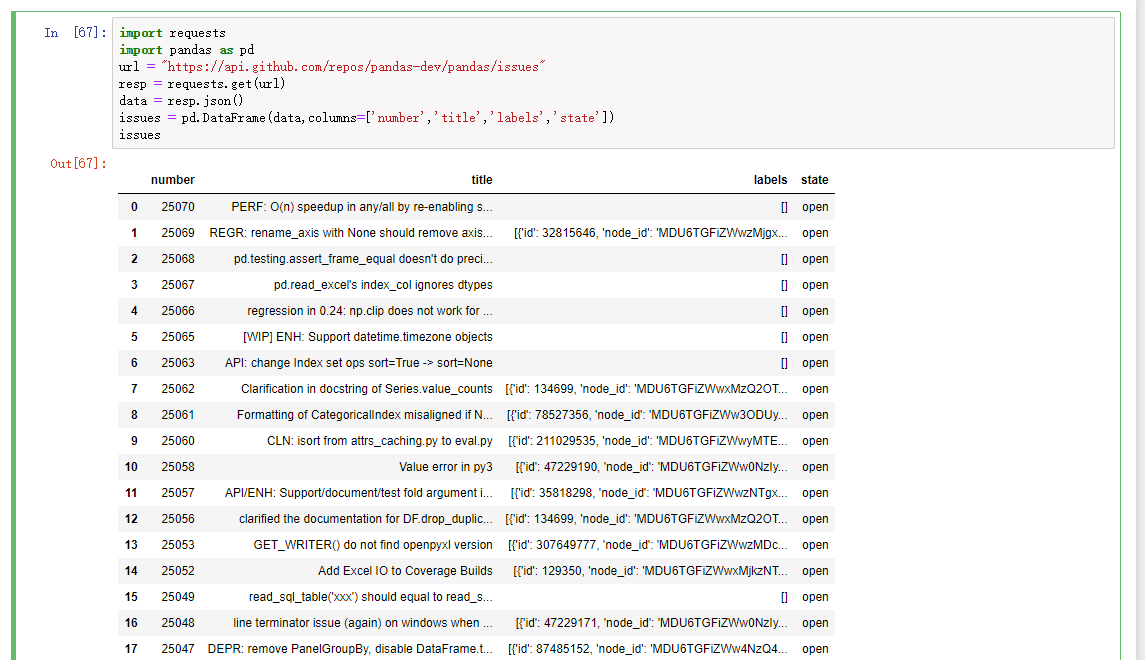## 4.操作数据库

### （1）导出数据

import pandas as pd
from sqlalchemy import create_engine
# import pymysql

# 创建数据库连接
conn = create_engine("mysql+pymysql://root:123@localhost:3306/luffy")

sql = "select * from luffy_banner;"
# # 通过sql语句以及连接查询数据库，最终返回的结果是一个DataFrame数组
df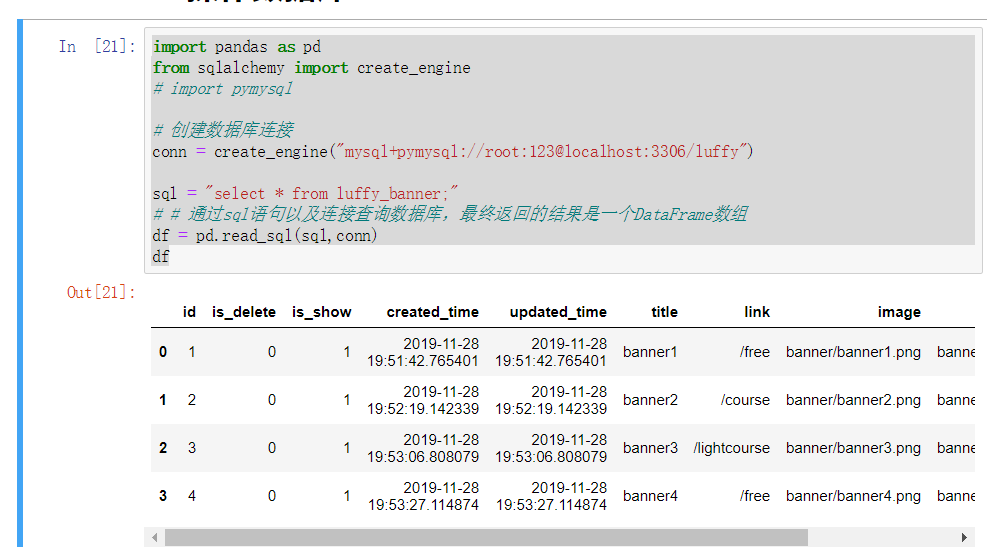### （2）导入数据

import pandas as pd
from sqlalchemy import create_engine
import pymysql

# 创建数据库连接
conn = create_engine("mysql+pymysql://root:123@localhost:3306/luffy")

# key为列，键为值
data = pd.DataFrame({'id':[1,2,3],
'name':['贾玲','沈腾','马丽'],
# 第一个参数：新建的表名；第二个：数据库连接
data.to_sql('pd_test1',conn,index = True)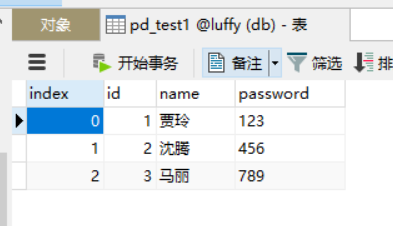# 三、数据分组和聚合

## 1. 分组（GroupBy机制）

pandas对象（无论Series、DataFrame还是其他的什么）当中的数据会根据提供的一个或者多个键被拆分为多组，拆分操作实在对象的特定轴上执行分，就比如DataFrame可以在他的行上或者列上进行分组，然后将一个函数应用到各个分组上并产生一个新的值。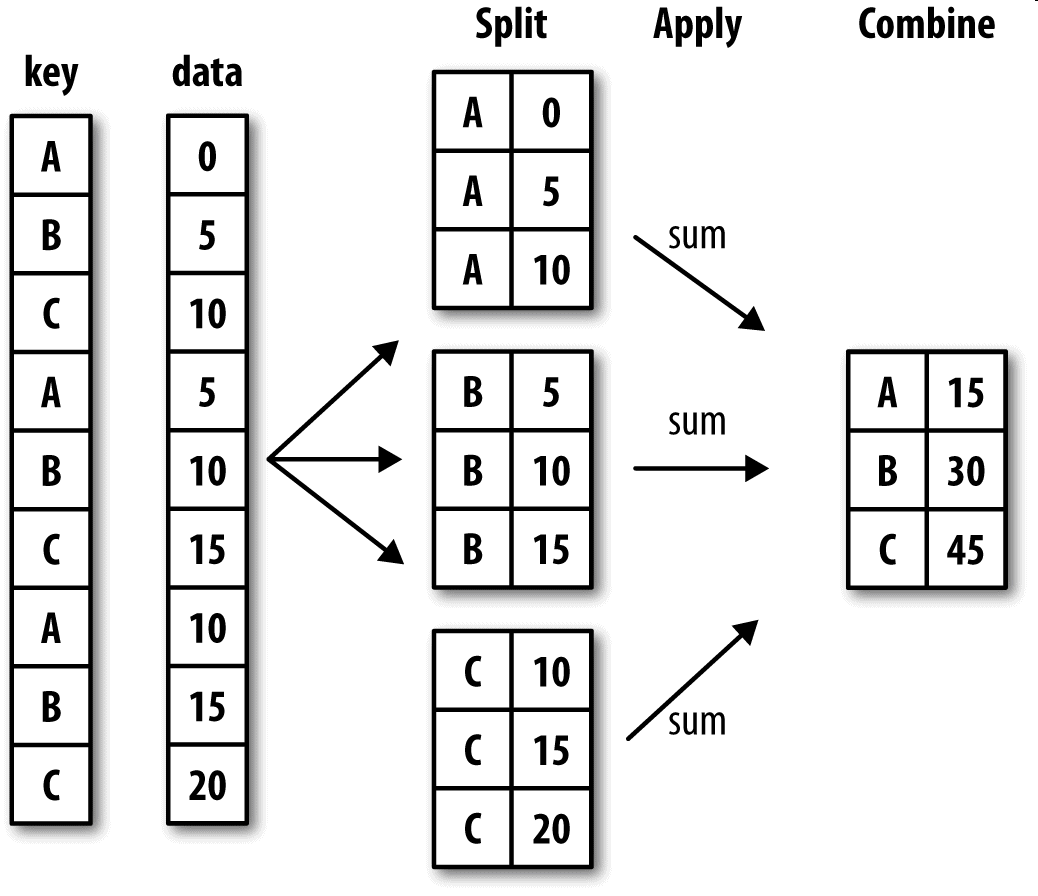• 列表或者数组，长度要与待分组的轴一样
• 表示DataFrame某个列名的值。
• 字典或Series，给出待分组轴上的值与分组名之间的对应关系
• 函数，用于处理轴索引或者索引中的各个标签

df = pd.DataFrame({'key1':['x','x','y','y','x',
'key2':['one','two','one',',two','one'],
'data1':np.random.randn(5),
'data2':np.random.randn(5)})
df

>   key1    key2    data1   data2
0   x   one 0.951762    1.632336
1   x   two -0.369843   0.602261
2   y   one 1.512005    1.331759
3   y   two 1.383214    1.025692
4   x   one -0.475737   -1.182826

1.访问data1，并根据key1调用groupby
f1 = df['data1'].groupby(df['key'])
print(f1)
结果如下：
<pandas.core.groupby.generic.SeriesGroupBy object at 0x000002906F7EC780>

2.上述运行是没有进行任何计算的，但是我们想要的中间数据已经拿到了，接下来，就可以调用groupby进行任何计算了
f1.mean() # 调用mean函数求出平均值
结果如下：
key1
x   -0.023235
y   -0.616363
Name: data1, dtype: float64

3.以上数据经过分组键（一个Series数组）进行了聚合，产生了一个新的Series，索引就是key1列中的唯一值。这些索引名称就为key1.接下来就尝试一次将多个数据的列表传进来
f2 = df['data1'].groupby([df['key1'],df['key2']])
f2.mean()
结果如下：
key1  key2
x     one     0.407317
two    -0.884339
y     two   -0.940015
one    -0.292710
Name: data1, dtype: float64

4.传入多个数据之后，就会发现，得到的数据具有一个层次化的索引，key1对应的x/y；key2对应的noe/two
f2.mean().unstack()  # 通过unstack方法就可以让索引不堆叠在一起了
结果如下：
key2	one	two
key1
x	0.552454	-0.962275
y	1.244774	0.183788


• 分组键可以是任意长度的数组
• 分组时，会与不是数组数据的列会从结果中排除，例如key1、key2这样的列
• Groupby的size方法，返回一个含有分组大小的Series
# 以上面的f2测试
f2.size()
> key1  key2
x     one     2
two     1
y     one     1
two     1
Name: data1, dtype: int64


## 2. 聚合（组内应用某个函数）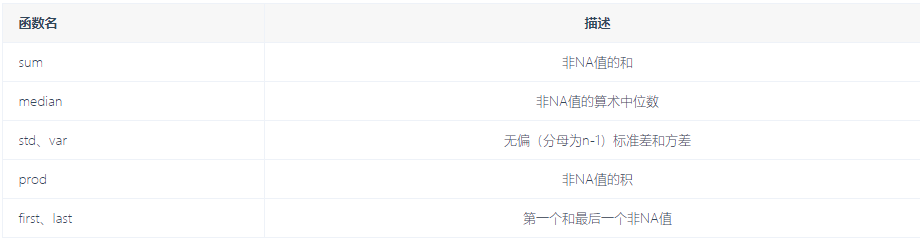df = pd.DataFrame({'key1':['x','x','y','y','x'],
'key2':['one','two','one','two','one'],
'data1':np.random.randn(5),
'data2':np.random.randn(5)})
f1 = df['data1'].groupby(df['key1'])

# 自定义的函数
def peak_to_peak(arr):
return arr.max() - arr.min()

# 使用自定义的聚合函数，需要将其传入aggregate或者agg方法当中
f1.aggregate(peak_to_peak)

key1
x    3.378482
y    1.951752
Name: data1, dtype: float64


f1.agg(['mean','std'])

mean    std
key1
x   -0.856065   0.554386
y   -0.412916   0.214939


## 3. apply

Groupby当中自由度最高的方法就是apply，它会将待处理的对象拆分为多个片段，然后各个片段分别调用传入的函数，最后将他们组合在一起。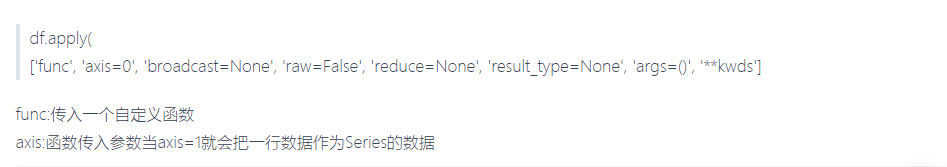# 分析欧洲杯和欧洲冠军联赛决赛名单
import pandas as pd

url="https://en.wikipedia.org/wiki/List_of_European_Cup_and_UEFA_Champions_League_finals"
a1 = eu_champions    # 取出决赛名单
a1.columns = a1.loc  # 使用第一行的数据替换默认的横向索引
a1.drop(0,inplace=True)  # 将第一行的数据删除
a1.drop('#',axis=1,inplace=True)  # 将以#为列名的那一列删除
a1.columns=['Season', 'Nation', 'Winners', 'Score', 'Runners_up', 'Runners_up_Nation', 'Venue','Attendance']  # 设置列名

a1.tail()  # 查看后五行数据
a1.drop([64,65],inplace=True)  # 删除其中的缺失行以及无用行
a1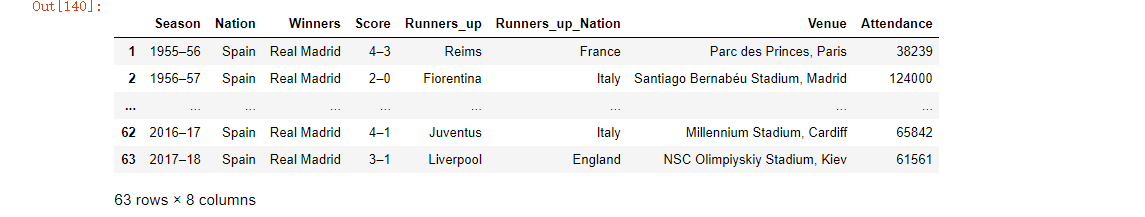# 先自定义一个函数
def top(df,n=3,column='Attendance'):
return df.sort_values(by=column)[-n:]
top(a1,n=3)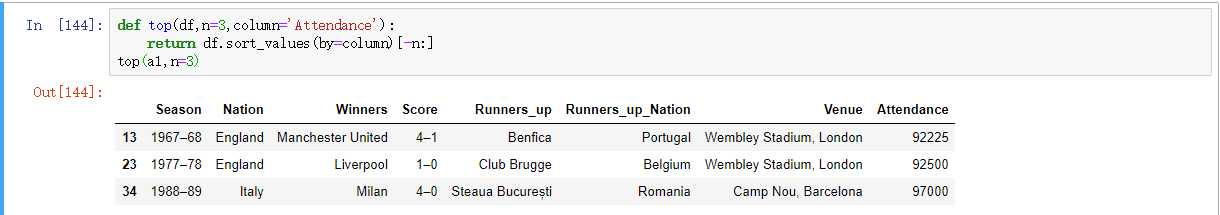a1.groupby('Nation').apply(top)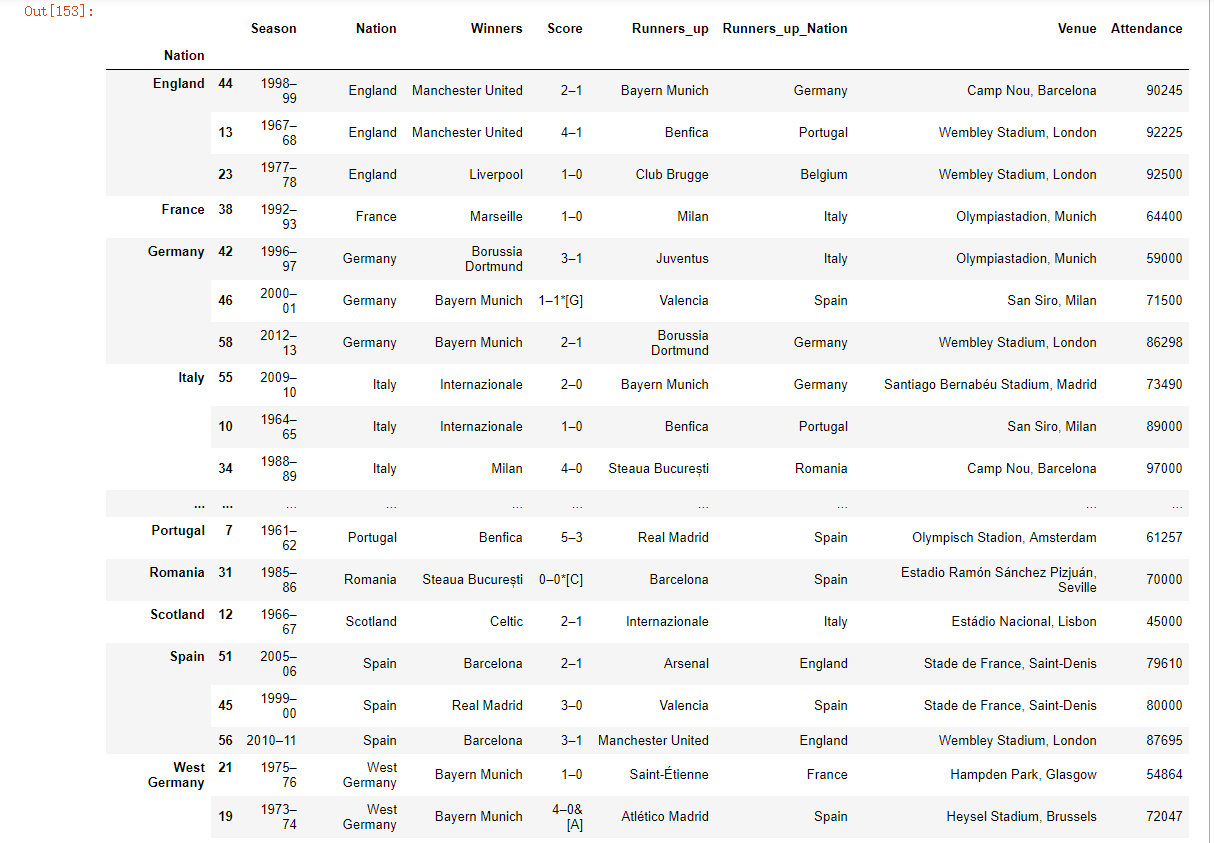# 四、pandas其他常用方法

pandas常用方-法（使用Series和DataFrame）

• mean(axis=0,skipna=False)
• sum(axis=1)
• sort_index(axis,···ascending) 按行或列索引排序
• sort_value(by,axis,ascending)按值排序
• apply(func,axis=0)将自定义函数应用在各行或各列上，func可反倒会标量或者Series
• applymap(func)将函数应用在DataFrame各个元素上
• map(func)将函数应用在Series各个元素上
posted @ 2020-02-16 14:49  chanyuli  阅读(401)  评论(0编辑  收藏